# Socio module

Virtual society | Socio-Module | Virus spread | Literature | Version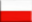Data | Model | Results

## General

In this area only some statistics were performed based on the virtual society model developed in the Virtual Society section.

Below are some basic numbers and statistical information concerning Poland.

## Statistical Poland

Population and the number of women and, as well as the number of households are given in Tab.1.

 Population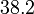$38.2$ x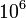$10^6$ Households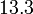$13.3$ x$10^6$ Women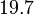$19.7$ x$10^6$ Men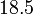$18.5$ x$10^6$

The administrative hierarchy of Poland is the following. The highest level belongs to voivodeship, which, following the European Nomenclature of Territorial Units for Statistics (NUTS) is classified as NUTS-2. There are 16 voivodeships in Poland. Second level constitute about 380 poviats (NUTS-4). The lowest level in the NUTS hierarchy is a commune (NUTS-1). There are about 2500 communes in Poland. There are several types of communes, but basically only three appear in Poland:

• urban/city communes (areas of larger cities)
• village/rural communes (area comprising several villages)
• city-village (urban-rural/mixed) (area comprising one smaller city and several villages)

The distribution of these 3 types of communes in Poland is presented in Fig.1. One can see that the total area of city communes is order of magnitude smaller than the total area of rural communes and about six times smaller than the total area of mixed communes. The corresponding numbers are given in Tab.1.

Commune type Population Area [km2] Population density [km-2]
urban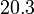$20.3$ x$10^6$ 15307 1328
rural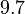$9.7$ x$10^6$ 198457 49
urban-rural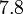$7.8$ x$10^6$ 99586 79

Most of the results presented here will be given in communal or poviat resolution.

 Primary school pupils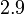$2.9$ x$10^6$ Schools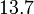$13.7$ x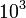$10^3$ Secondary school students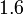$1.6$ x$10^6$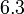$6.3$ x$10^3$ College students$2.5$ x$10^6$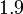$1.9$ x$10^3$ Employed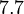$7.7$ x$10^6$ Workplaces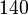$140$ x$10^3$